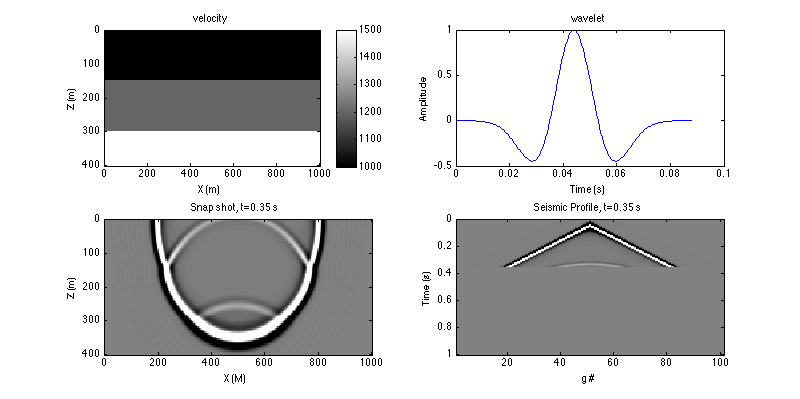# CSIM Wave-equation Series Lab

By Xin Wang (xin.wang@kaust.edu.sa), Bldg 1, 3139-WS13, 808-0386

### Part 1: TDFD solver to acoustic wave equation labCSIM Wave equation Series Lab Part 1: TDFD solver to acoustic wave equation lab PART 2: Reverse time migration lab PART 3: Born modeling and adjoint test lab PART 4: Wavepath lab; Objective: Learn and run the time domain finite difference (TDFD) solver to the 2D acoustic wave equation. Procedure: Define a 3 layer velocity model, and the model parameters; vel=[repmat(1000,[1,30]), repmat(1200,[1,30]), repmat(1500,[1,21])]; vel=repmat(vel',[1 201]); [nz,nx]=size(vel); dx=5; x = (0:nx-1)*dx; z = (0:nz-1)*dx; Define the source and receiver geometry; sx = (nx-1)/2*dx; sz = 0; gx=(0:2:(nx-1))*dx; gz=zeros(size(gx)); Setup FD parameters; nbc=40; nt=2001; dt=0.0005;isFS=false; Setup the source wavelet; freq=25; s=ricker(freq,dt); Plot the velocity and wavelet; figure(1);set(gcf,'position',[0 0 800 400]);subplot(221);imagesc(x,z,vel);colorbar; xlabel('X (m)'); ylabel('Z (m)'); title('velocity'); figure(1);subplot(222);plot((0:numel(s)-1)*dt,s); xlabel('Time (s)'); ylabel('Amplitude');title('wavelet'); Run the modeling code, watch the movie of wave propagation, and plot the seismic data; tic;seis=a2d_mod_abc28_snapshot(vel,nbc,dx,nt,dt,s,sx,sz,gx,gz,isFS);toc; Reminder: For all labs, you can copy the bold line command to a single script, and run the scripts to generate the same results. References: Seismic Inversion, Gerard T. Schuster